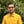Trusted answers to developer questions
Trusted Answers to Developer Questions

Related Tags

d
math
tanh
communitycreator

# What is tanh() in D?Harris Amjad

Grokking Modern System Design Interview for Engineers & Managers

Ace your System Design Interview and take your career to the next level. Learn to handle the design of applications like Netflix, Quora, Facebook, Uber, and many more in a 45-min interview. Learn the RESHADED framework for architecting web-scale applications by determining requirements, constraints, and assumptions before diving into a step-by-step design process.

The tanh() function in D returns a number’s hyperbolic tangent.

Figure 1 shows the mathematical representation of the tanh() function:

Figure 1: Mathematical representation of the hyperbolic tangent function

Note: We need to import std.math in our code to use the tanh() function. We can import it like this:
import std.math.

### Syntax

tanh(num)


### Parameter

This function requires a number representing an angle in radians as a parameter.

To convert degrees to radians, use:

radians = degrees * ( PI / 180.0 )


### Return value

tanh() returns the hyperbolic tangent of a number (radians) that is set as a parameter.

### Example

The code below shows the use of the tanh() function in D:

import core.stdc.stdio;import std.stdio;//header required for functionimport std.math;int main(){     //positive number in radians    writeln("The value of tanh(2.3) ", tanh(2.3));    // negative number in radians    writeln("The value of tanh(-2.3) ", tanh(-2.3));    //converting the degrees angle into radians and then applying tanh()    // degrees = 45.0    // PI = 3.14159265    // result first converts degrees to radians then apply tanh    double result=tanh(45.0 * (PI / 180.0));    writeln("The value of tanh(45.0 * (PI / 180.0)) ", result);        //exceptional output    writeln ("The value of tanh(real.infinity) : ",tanh(real.infinity));    writeln ("The value of tanh(-real.infinity) : ",tanh(-real.infinity));    writeln ("The value of tanh(real.nan) : ",tanh(real.nan));    writeln ("The value of tanh(-real.nan) : ",tanh(-real.nan));        return 0;}

### Explanation

Line 4: We add the header std.math required for the tanh() function.

Line 9: We calculate the hyperbolic tan of positive numbers in radians using tanh().

Line 12: We calculate the hyperbolic tan of a negative number in radians using tanh().

Line 18: We convert the degrees into radians and then apply the tanh() function. The variable result first converts degrees to radians and then applies tanh().

Line 21 onwards: We calculate the hyperbolic tan of exceptional numbers using tanh()

RELATED TAGS

d
math
tanh
communitycreator

CONTRIBUTORHarris Amjad

Grokking Modern System Design Interview for Engineers & Managers

Ace your System Design Interview and take your career to the next level. Learn to handle the design of applications like Netflix, Quora, Facebook, Uber, and many more in a 45-min interview. Learn the RESHADED framework for architecting web-scale applications by determining requirements, constraints, and assumptions before diving into a step-by-step design process.

Keep Exploring

Learn in-demand tech skills in half the time